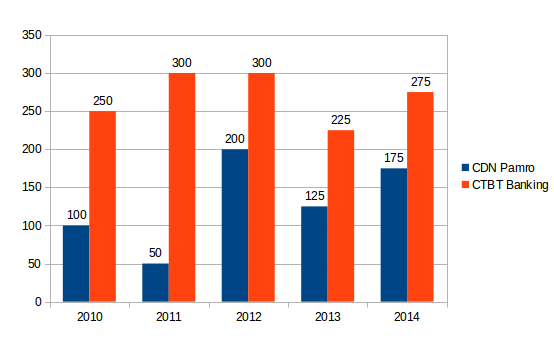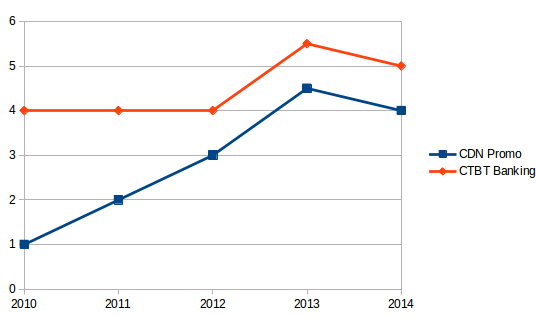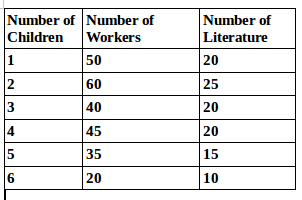## Data Interpretation For SBI PO : Set – 54

D.1-5) Study the following two graphs carefully and answer the questions that follow them.

The bar chart given below shows the gross loan amounts given by two banks CDN Pamro Bank and CTBT Banking Corporation over six years.The line graph below this bar chart shows the average percentage of seed capital (as a percentage of the loan granted in given year) given by the clients of the bank to awail the loan(in each year). Now, answer the following questions.1) In the year 2012, the net loan issued by CTBT Banking was more than that issued by CDN Pamro by what amount?

a) 100 Crore

b) 99 Crore

C) 291 Crore

d) 192 crore

e) 199 Crore

b)

For year 2012 (refer bar Chart)

Gross loans issued by CDN Pamro =Rs. 200 Crore

Gross loans issued by CTBT Banking = Rs. 300 Crore

For year 2012(refer graph)

Average Percentage of seed capital (CDN Pamro)=4%

Average Percentage of seed capital (CTBT Banking) = 3%

If the gross loan issued is Rs.100 and the percentage of seed capital in it is 10% ,it means that the net loan issued is Rs.100-100*10%=Rs.90 Use this tenet to solve the questions.

So, for year 2012, Seed capital received by CDN Pamro = 200*4% = 200*0.04 = Rs.8 crore

Net loan issued = Gross Loan – Seed Capital = 200-8 = Rs.192 Crore

For year 2012, seed capital received by CTBT Banking = 300*3%= 300*0.3 = Rs.9 crore

Net loan issue = 300-9 = 291 Crore

The net loan issued by CTBT Banking was more than the net loan issued by CDN Pamro by an amount (291-192)=Rs.99 Crore

2) If the loans for the year 2014 were mortaged (through a mortage agreement) by CTBT banking and were to the tune of Rs. 171.25 crore, then what is the amount of loans that was not mortgaged through mortgage agreements?

a) 275 crore

b) 90 Crore

c) 190 crore

d) 121 Crore

e) None of these

b)

For the year 2014:

Gross loans issued by CTBT banking = Rs. 275 Crore

For the year 2014

Seed Capital percentage in CTBT Banking = 5%

Hence, seed Capital = 275 * 0.5% = 275*0.05= 13.75Crore

Net loan = 275 – 13.75= Rs.216.25 Crore

Secured loans (through mortgage agreement Rs.171.25 crore)

Loans for 2014 that are not secured through mortgage agreements for CTBT Banking = 261.25- 171.25 = 90 crore

3) What is the difference between the seed capital collected by CDN Pamro in 2011 and the the seed Capital collected by CTBT Banking in 2013?

a) 13.5 Crore

b) 1 Crore

c) 14.5 Crore

d) 2.1 Crore

e) 12.5 Crore

e)

e) In 2011, the seed capital percentage of CDN Pamro was 2%

Hence Seed Capital = 50 *0.02= 1 crore

In 2013, the loans issued by CTBT Banking were Rs.225 Crore

In 2013, the seed capital percentage of CTBT Banking was 6%

Hence Seed Capital = 50 * 0.02 = 1 crore

In 2013, the loans issued by CTBT Banking were Rs.225 Crore

In 2013, the seed capital percentage of CTBT Banking was 6%

Hence seed Capital = 225 *0.06=Rs.13.5 Crore

Difference = 13.50-1.00 = 12.5 crore

4) What was the total amount of seed capital collected by CTBT banking in the years 2011 and 2012 combined?

a) 12 Crore

b) 9 Crore

c) 30 Crore

d) 21 Crore

e) 20 Crore

d)

For the years 2011 and 2012, the loans issued by CTBT Banking were of the same amount i.e) 300 crore

For 2011 seed capital was 3% of loan amount for CTBT Banking

Hence Seed Capital for 2011 = 300 * 0.04 = 12 crore

Seed capital for 2012 = 300 * 0.03 = 9 crore

Total Seed capital collected in 2011 and 2012 by CTBT Banking = 12+9 = 21 crore

5) What was the difference of seed capital collected by CDN Pamro in 2011 and 2012 and seed Capital Collected by CTBT banking in 2014?

a) 13.75 Crore

b) 4 Crore

c) 9.75 Crore

d) 5.75 Crore

e) 5.25 Crore

c)

For 2010 , loan amount issued by CDN Pamro = 100 Crore

For 2020 , percentage of seed capital (CDN Pamro) = 4%

Hence Seed Capital = 100*0.04 = 4 crore

For 2014, loan amounts issued by CTBT Banking = Rs. 275 Crore

For 2014 percentage of seed capital (CTBT Banking) = 5%

Hence Seed Capital = 275 * 0.05 = Rs.13.75 Crore

Difference = 13.75 – 4 = 9.75 Crore

D.6-10) Study the following graph carefully and answer the questions that follow it.

The following graph shows three curves of data for Avicorp Bank Ltd, There are three kinds of deposits CC , CA, and SB6) The total number of workers in the factory is

a) 300

b) 250

c) 200

d) 175

e) 150

b)

50+60+40+45+35+20= 250

7) The total number of children of all the workers is

a) 765

b) 750

c) 745

d) 725

e) 715

a)

a)  50 * 1 +60 * 2+40 * 3+45 * 4+35 * 5+20 * 6 = 50+120+120+180+175+120 = 765

8) The total number of literature workers is

a) 95

b) 100

c) 110

d) 120

e) 135

c)

20+25+20+20+15+10= 110

9) The number of illiterate workers with 3 or more children is

a) 120

b) 180

c) 85

d) 65

e) 75

d)

The number of literature workers who have less than 4 children = 20+25+15+10=65

10) The number of literate workers with less than children is

a) 65

b) 85

c) 90

d) 135

e) 145